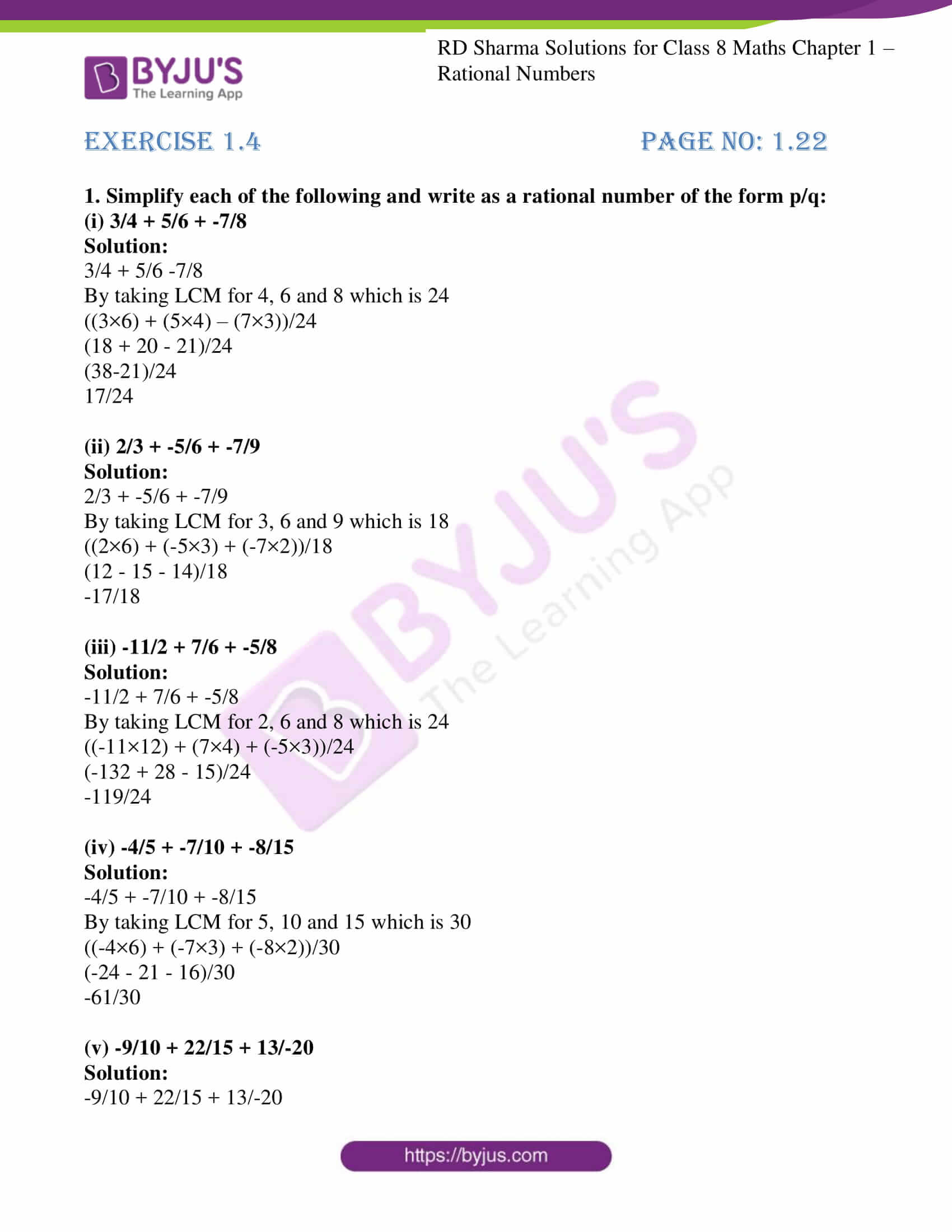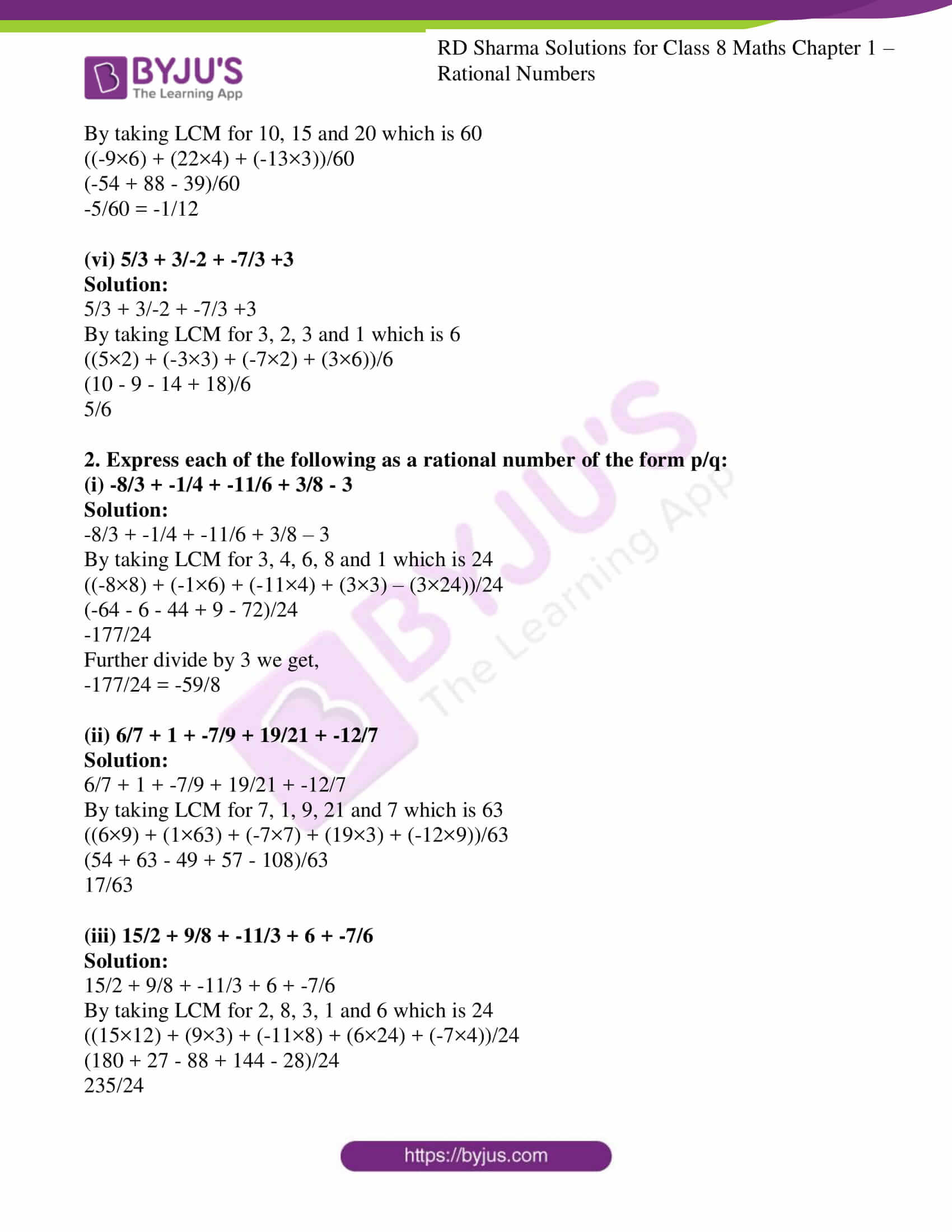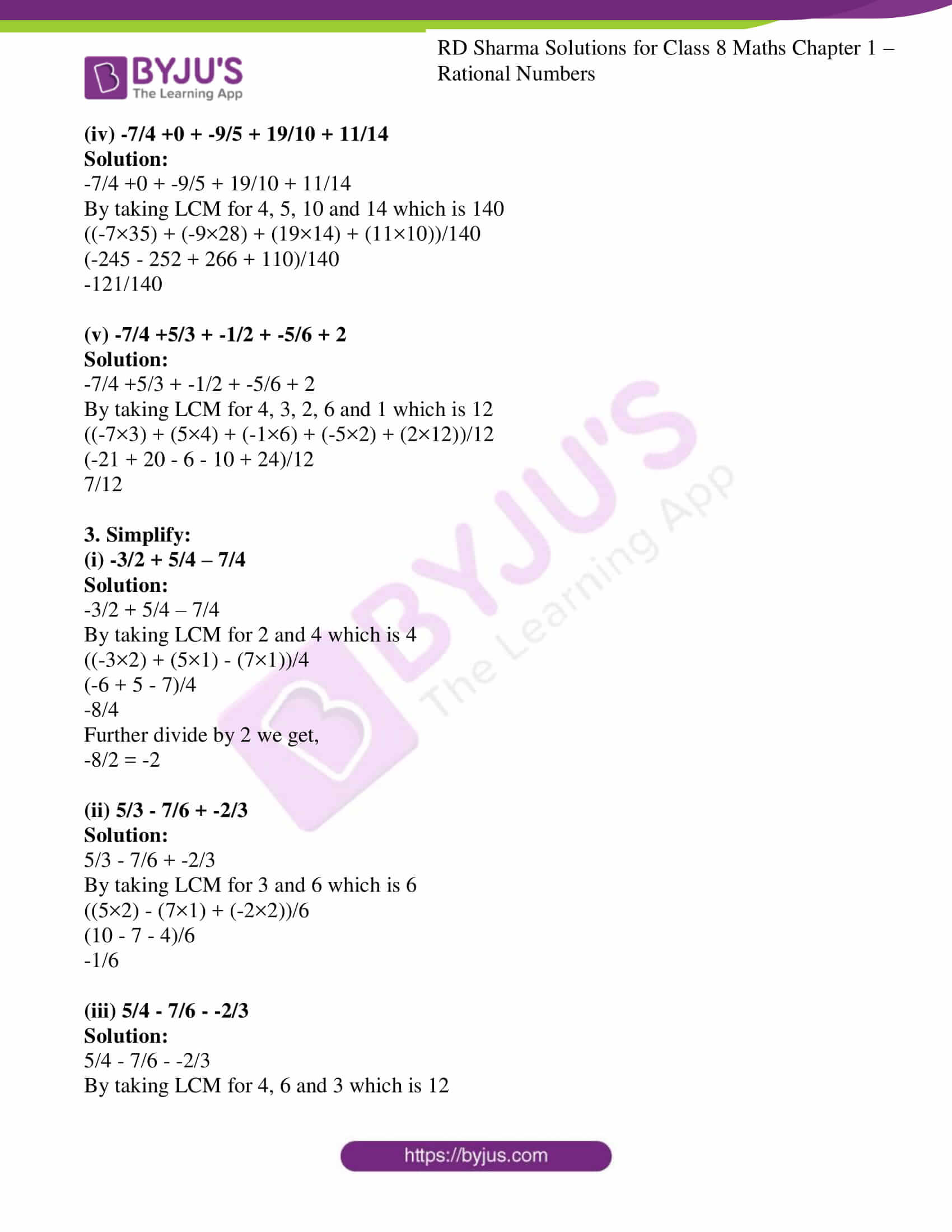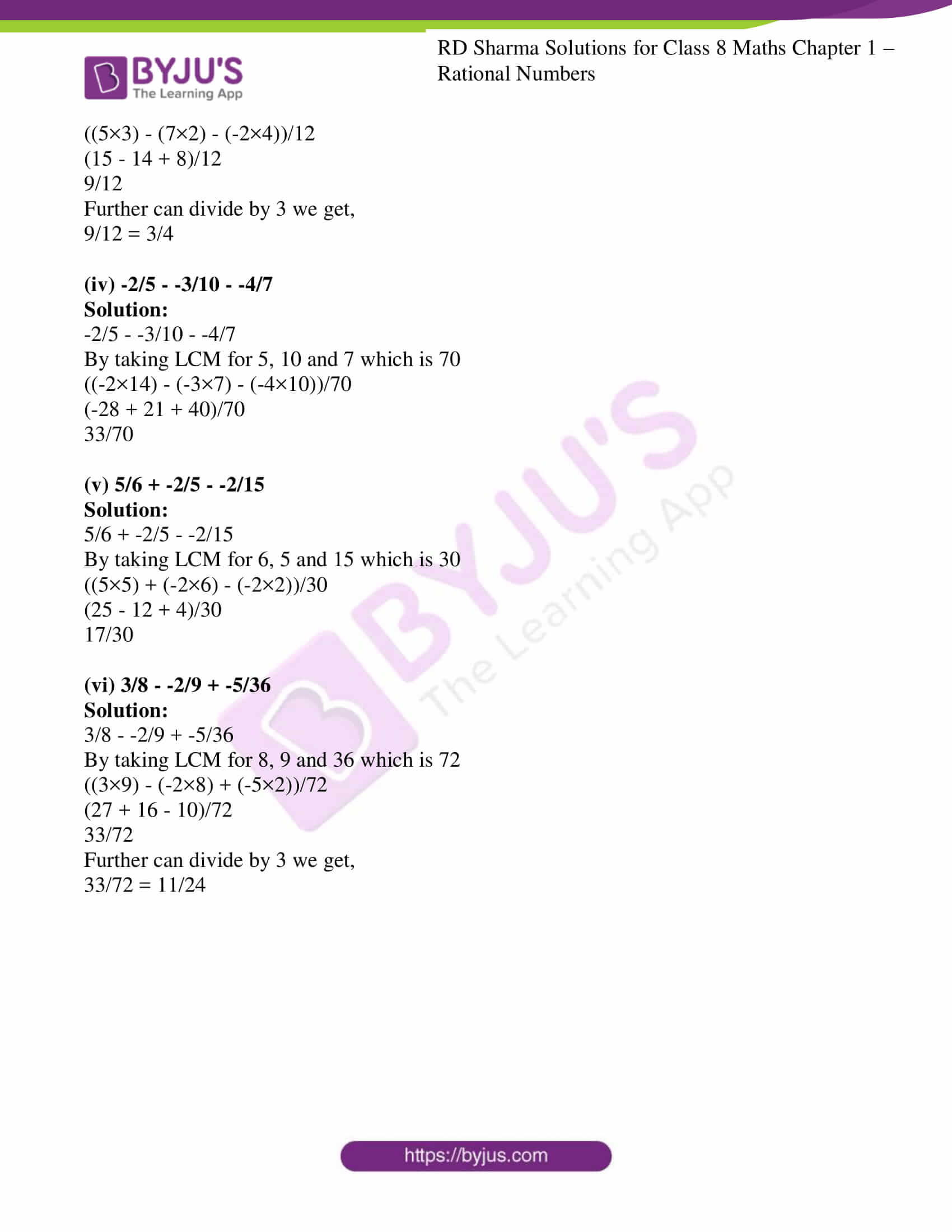# RD Sharma Solutions for Class 8 Maths Chapter 1 - Rational Numbers Exercise 1.4

In Exercise 1.4 of RD Sharma Class 8 Maths Chapter 1 Rational numbers, we shall discuss problems based on the simplification of expressions involving addition and subtraction of rational numbers. RD Sharma Solutions Class 8 are solved by our experts in order to help students in learning the concepts from the textbook effortlessly. Students can easily download the pdf from the links provided below.

## Download PDF of RD Sharma Solutions for Class 8 Maths Exercise 1.4 Chapter 1 Rational Numbers### Access Answers to RD Sharma Solutions for Class 8 Maths Exercise 1.4 Chapter 1 Rational Numbers

1. Simplify each of the following and write as a rational number of the form p/q:

(i) 3/4 + 5/6 + -7/8

Solution:

3/4 + 5/6 -7/8

By taking LCM for 4, 6 and 8 which is 24

((3×6) + (5×4) – (7×3))/24

(18 + 20 – 21)/24

(38-21)/24

17/24

(ii) 2/3 + -5/6 + -7/9

Solution:

2/3 + -5/6 + -7/9

By taking LCM for 3, 6 and 9 which is 18

((2×6) + (-5×3) + (-7×2))/18

(12 – 15 – 14)/18

-17/18

(iii) -11/2 + 7/6 + -5/8

Solution:

-11/2 + 7/6 + -5/8

By taking LCM for 2, 6 and 8 which is 24

((-11×12) + (7×4) + (-5×3))/24

(-132 + 28 – 15)/24

-119/24

(iv) -4/5 + -7/10 + -8/15

Solution:

-4/5 + -7/10 + -8/15

By taking LCM for 5, 10 and 15 which is 30

((-4×6) + (-7×3) + (-8×2))/30

(-24 – 21 – 16)/30

-61/30

(v) -9/10 + 22/15 + 13/-20

Solution:

-9/10 + 22/15 + 13/-20

By taking LCM for 10, 15 and 20 which is 60

((-9×6) + (22×4) + (-13×3))/60

(-54 + 88 – 39)/60

-5/60 = -1/12

(vi) 5/3 + 3/-2 + -7/3 +3

Solution:

5/3 + 3/-2 + -7/3 +3

By taking LCM for 3, 2, 3 and 1 which is 6

((5×2) + (-3×3) + (-7×2) + (3×6))/6

(10 – 9 – 14 + 18)/6

5/6

2. Express each of the following as a rational number of the form p/q:

(i) -8/3 + -1/4 + -11/6 + 3/8 – 3

Solution:

-8/3 + -1/4 + -11/6 + 3/8 – 3

By taking LCM for 3, 4, 6, 8 and 1 which is 24

((-8×8) + (-1×6) + (-11×4) + (3×3) – (3×24))/24

(-64 – 6 – 44 + 9 – 72)/24

-177/24

Further divide by 3 we get,

-177/24 = -59/8

(ii) 6/7 + 1 + -7/9 + 19/21 + -12/7

Solution:

6/7 + 1 + -7/9 + 19/21 + -12/7

By taking LCM for 7, 1, 9, 21 and 7 which is 63

((6×9) + (1×63) + (-7×7) + (19×3) + (-12×9))/63

(54 + 63 – 49 + 57 – 108)/63

17/63

(iii) 15/2 + 9/8 + -11/3 + 6 + -7/6

Solution:

15/2 + 9/8 + -11/3 + 6 + -7/6

By taking LCM for 2, 8, 3, 1 and 6 which is 24

((15×12) + (9×3) + (-11×8) + (6×24) + (-7×4))/24

(180 + 27 – 88 + 144 – 28)/24

235/24

(iv) -7/4 +0 + -9/5 + 19/10 + 11/14

Solution:

-7/4 +0 + -9/5 + 19/10 + 11/14

By taking LCM for 4, 5, 10 and 14 which is 140

((-7×35) + (-9×28) + (19×14) + (11×10))/140

(-245 – 252 + 266 + 110)/140

-121/140

(v) -7/4 +5/3 + -1/2 + -5/6 + 2

Solution:

-7/4 +5/3 + -1/2 + -5/6 + 2

By taking LCM for 4, 3, 2, 6 and 1 which is 12

((-7×3) + (5×4) + (-1×6) + (-5×2) + (2×12))/12

(-21 + 20 – 6 – 10 + 24)/12

7/12

3. Simplify:

(i) -3/2 + 5/4 – 7/4

Solution:

-3/2 + 5/4 – 7/4

By taking LCM for 2 and 4 which is 4

((-3×2) + (5×1) – (7×1))/4

(-6 + 5 – 7)/4

-8/4

Further divide by 2 we get,

-8/2 = -2

(ii) 5/3 – 7/6 + -2/3

Solution:

5/3 – 7/6 + -2/3

By taking LCM for 3 and 6 which is 6

((5×2) – (7×1) + (-2×2))/6

(10 – 7 – 4)/6

-1/6

(iii) 5/4 – 7/6 – -2/3

Solution:

5/4 – 7/6 – -2/3

By taking LCM for 4, 6 and 3 which is 12

((5×3) – (7×2) – (-2×4))/12

(15 – 14 + 8)/12

9/12

Further can divide by 3 we get,

9/12 = 3/4

(iv) -2/5 – -3/10 – -4/7

Solution:

-2/5 – -3/10 – -4/7

By taking LCM for 5, 10 and 7 which is 70

((-2×14) – (-3×7) – (-4×10))/70

(-28 + 21 + 40)/70

33/70

(v) 5/6 + -2/5 – -2/15

Solution:

5/6 + -2/5 – -2/15

By taking LCM for 6, 5 and 15 which is 30

((5×5) + (-2×6) – (-2×2))/30

(25 – 12 + 4)/30

17/30

(vi) 3/8 – -2/9 + -5/36

Solution:

3/8 – -2/9 + -5/36

By taking LCM for 8, 9 and 36 which is 72

((3×9) – (-2×8) + (-5×2))/72

(27 + 16 – 10)/72

33/72

Further can divide by 3 we get,

33/72 = 11/24

## RD Sharma Solutions for Class 8 Maths Exercise 1.4 Chapter 1 Rational Numbers

Class 8 Maths Chapter 1 Rational Numbers Exercise 1.4 is based on the simplification of expressions involving addition and subtraction of rational numbers. These solutions are prepared by experienced faculty after vast research is conducted on each concept. The exercise-wise solutions are explained in a simple and easily understandable language which helps students in their exam preparation. Download free RD Sharma Solutions Chapter 1 in PDF format which provides answers to all the questions.